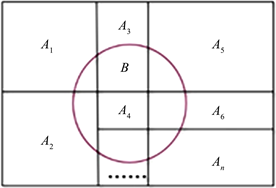﻿ 全概率公式的教学方法的思考

# 全概率公式的教学方法的思考Thinking about Teaching Method of Total Probability Formula

Abstract: The total probability formula is a key and difficult content in the teaching of probability theory and mathematical statistics. A new teaching design for the teaching process of the total probability formula is proposed, in which we explain the relationship between the formula and classical probability and tell the method of looking for the division of sample space through examples. Finally, the total probability formula is applied to the investigation of sensitive problems.

1. 引言

2. 教学过程

2.1. 概念的引入Figure 1. Decomposition of complex event B

2.2. 全概率公式的证明

1) ${A}_{i}{A}_{j}=\varphi ,\text{\hspace{0.17em}}\text{\hspace{0.17em}}i\ne j,\text{\hspace{0.17em}}\text{\hspace{0.17em}}i,j=1,2,\cdots ,n$ ；2) $\underset{i=1}{\overset{n}{\cup }}{A}_{i}=S$

$P\left(B\right)=\underset{i=1}{\overset{n}{\sum }}P\left({A}_{i}\right)P\left(B|{A}_{i}\right)$ (1)

$B=B\cap S=B\cap \left({A}_{1}\cup {A}_{2}\cup \cdots \cup {A}_{n}\right)=B{A}_{1}\cup B{A}_{2}\cup \cdots \cup B{A}_{n}$

$B{A}_{\text{1}},B{A}_{\text{2}},\cdots ,B{A}_{n}$ 互不相容，由概率的有限可加性得

$P\left(B\right)=P\left(\underset{i=1}{\overset{n}{\cup }}B{A}_{i}\right)=\underset{i=1}{\overset{n}{\sum }}P\left(B{A}_{i}\right)$

$P\left(B{A}_{i}\right)=P\left({A}_{i}\right)P\left(B|{A}_{i}\right)$

$P\left(B\right)=\underset{i=1}{\overset{n}{\sum }}P\left({A}_{i}\right)P\left(B|{A}_{i}\right)$

2.3. 例题

$P\left(B\right)=\underset{i=1}{\overset{n}{\sum }}P\left({A}_{i}\right)P\left(B|{A}_{i}\right)=\frac{1}{3}×\frac{1}{5}+\frac{1}{3}×\frac{2}{5}+\frac{1}{3}×1=\frac{8}{15}$.

$P\left({A}_{\text{1}}\right)=\text{0}\text{.6},\text{\hspace{0.17em}}\text{\hspace{0.17em}}P\left({A}_{\text{2}}\right)=\text{0}\text{.4},\text{\hspace{0.17em}}\text{\hspace{0.17em}}P\left(B|{A}_{\text{1}}\right)=\text{0}\text{.99},\text{\hspace{0.17em}}\text{\hspace{0.17em}}P\left(B|{A}_{\text{2}}\right)=\text{0}\text{.02}$

$P\left(B\right)=P\left({A}_{\text{1}}\right)P\left(B|{A}_{\text{1}}\right)+P\left({A}_{2}\right)P\left(B|{A}_{\text{2}}\right)=0.6×0.99+0.4×0.02=0.602$.

2.4. 对全概率公式的理解

2.5. 全概率公式的应用

B表示回答“是”，A1表示掷硬币正面朝上，A2表示掷硬币反面朝上；此时 $\text{\hspace{0.17em}}P\left(B|{A}_{\text{1}}\right)$ 就是我们关心的同性恋人群的比例， $P\left(B|{A}_{\text{2}}\right)$ 表示人群中电话号码最后一位为偶数的概率，是已知的。则由全概率公式

$P\left(B\right)=P\left({A}_{\text{1}}\right)P\left(B|{A}_{\text{1}}\right)+P\left({A}_{\text{2}}\right)P\left(B|{A}_{\text{2}}\right)=\frac{1}{2}P\left(B|{A}_{\text{1}}\right)+\frac{1}{2}P\left(B|{A}_{\text{2}}\right)$

3. 结论

 盛骤, 谢式千, 潘承毅. 概率论与数理统计[M]. 第四版. 北京: 高等教育出版, 2008.

 陈希孺. 概率论与数理统计[M]. 第一版. 合肥: 中国科学技术大学出版社, 2009.

 茆诗松, 程依明, 濮晓龙. 概率论与数理统计[M]. 第二版. 北京: 高等教育出版社, 2011.

 唐旭晖, 李冱岸, 段利霞. 全概率公式的推广与应用[J]. 高等数学研究, 2011, 14(4): 51-52.

Top